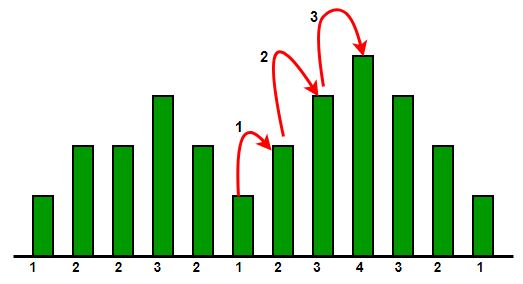Open in App
Not now

# Maximise consecutive steps one can put forward on roof to gain an increase in altitude

• Difficulty Level : Basic
• Last Updated : 15 Sep, 2022

Given the heights of consecutive buildings, find the maximum number of consecutive steps one can put forward such that he gains an increase in altitude while going from the roof of one building to the next adjacent one.Examples:

Input: arr[] = {1, 2, 2, 3, 2}
Output: 1
Explanation: Maximum consecutive steps from 1 to 2 OR  2 to 3

Input: arr[] = {1, 2, 3, 4}
Output: 3

## Consecutive steps to the rooftop by finding the longest increasing subarray:

To solve the problem follow the below idea:

This problem is basically a variation of the Longest increasing subarray problem

Follow the given steps to solve the problem:

• initialize count = 0
• initialize maximum = 0
• if arr[i] > a[i-1], then increment count
• else maximum = max(maximum, count) and set count equal to zero
• At the end return maximum = max(maximum, count)

Below is the implementation of the above approach:

## C++

 `// C++ code to find maximum` `// number of consecutive steps.` `#include ` `using` `namespace` `std;`   `// Function to count consecutive steps` `int` `find_consecutive_steps(``int` `arr[], ``int` `len)` `{` `    ``int` `count = 0;` `    ``int` `maximum = 0;`   `    ``for` `(``int` `index = 1; index < len; index++) {`   `        ``// count the number of consecutive` `        ``// increasing height building` `        ``if` `(arr[index] > arr[index - 1])` `            ``count++;` `        ``else` `{` `            ``maximum = max(maximum, count);` `            ``count = 0;` `        ``}` `    ``}`   `    ``return` `max(maximum, count);` `}`   `// Driver code` `int` `main()` `{` `    ``int` `arr[] = { 1, 2, 3, 4 };` `    ``int` `len = ``sizeof``(arr) / ``sizeof``(arr);`   `    ``// Function call` `    ``cout << find_consecutive_steps(arr, len);` `}`

## Java

 `// Java code to find maximum` `// number of consecutive steps.` `import` `java.io.*;`   `class` `GFG {`   `    ``// Function to count consecutive steps` `    ``static` `int` `find_consecutive_steps(``int` `arr[], ``int` `len)` `    ``{` `        ``int` `count = ``0``;` `        ``int` `maximum = ``0``;`   `        ``for` `(``int` `index = ``1``; index < len; index++) {`   `            ``// count the number of consecutive` `            ``// increasing height building` `            ``if` `(arr[index] > arr[index - ``1``])` `                ``count++;` `            ``else` `{` `                ``maximum = Math.max(maximum, count);` `                ``count = ``0``;` `            ``}` `        ``}`   `        ``return` `Math.max(maximum, count);` `    ``}`   `    ``// Driver code` `    ``public` `static` `void` `main(String[] args)` `    ``{`   `        ``int` `arr[] = { ``1``, ``2``, ``3``, ``4` `};` `        ``int` `len = arr.length;`   `        ``// Function call` `        ``System.out.println(` `            ``find_consecutive_steps(arr, len));` `    ``}` `}`   `// This code is contributed by Gitanjali.`

## Python3

 `# Python3 code to find maximum` `# number of consecutive steps` `import` `math`   `# Function to count consecutive steps`     `def` `find_consecutive_steps(arr, ``len``):`   `    ``count ``=` `0` `    ``maximum ``=` `0`   `    ``for` `index ``in` `range``(``1``, ``len``):`   `        ``# count the number of consecutive` `        ``# increasing height building` `        ``if` `(arr[index] > arr[index ``-` `1``]):` `            ``count ``+``=` `1`   `        ``else``:` `            ``maximum ``=` `max``(maximum, count)` `            ``count ``=` `0`   `    ``return` `max``(maximum, count)`     `# Driver code` `if` `__name__ ``=``=` `"__main__"``:` `    ``arr ``=` `[``1``, ``2``, ``3``, ``4``]` `    ``len` `=` `len``(arr)`   `    ``# Function call` `    ``print``(find_consecutive_steps(arr, ``len``))`     `# This code is contributed by Gitanjali.`

## C#

 `// C# code to find maximum` `// number of consecutive steps.` `using` `System;`   `class` `GFG {`   `    ``// Function to count consecutive steps` `    ``static` `int` `find_consecutive_steps(``int``[] arr, ``int` `len)` `    ``{` `        ``int` `count = 0;` `        ``int` `maximum = 0;`   `        ``for` `(``int` `index = 1; index < len; index++) {`   `            ``// count the number of consecutive` `            ``// increasing height building` `            ``if` `(arr[index] > arr[index - 1])` `                ``count++;` `            ``else` `{` `                ``maximum = Math.Max(maximum, count);` `                ``count = 0;` `            ``}` `        ``}`   `        ``return` `Math.Max(maximum, count);` `    ``}`   `    ``// Driver code` `    ``public` `static` `void` `Main()` `    ``{`   `        ``int``[] arr = { 1, 2, 3, 4 };` `        ``int` `len = arr.Length;`   `        ``// Function call` `        ``Console.WriteLine(find_consecutive_steps(arr, len));` `    ``}` `}`   `// This code is contributed by vt_m.`

## PHP

 ` ``\$arr``[``\$index` `- 1])` `            ``\$count``++;` `        ``else` `        ``{` `            ``\$maximum` `= max(``\$maximum``, ``\$count``);` `            ``\$count` `= 0;` `        ``}` `    ``}` `    `  `    ``return` `max(``\$maximum``, ``\$count``); ` `}`   `// Driver code` `\$arr` `= ``array``( 1, 2, 3, 4 );` `\$len` `= ``count``(``\$arr``);`   `// Function call` `echo` `find_consecutive_steps(``\$arr``, ``\$len``);`   `// This code is contributed by vt_m. ` `?>`

## Javascript

 ``

Output

`3`

Time Complexity: O(N)
Auxiliary Space: O(1)

My Personal Notes arrow_drop_up
Related Articles